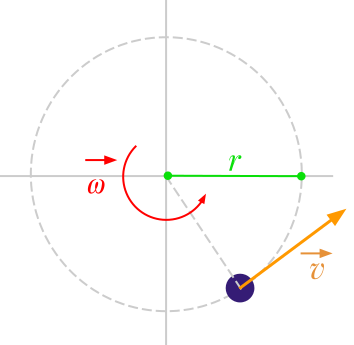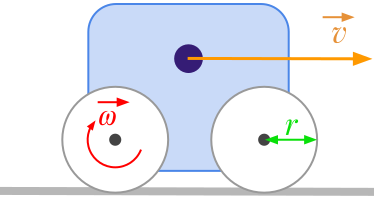Question #1055

# How to convert angular speed [rad/s] to linear velocity [m/s]?

Merged questionsThe formula to calculate the linear speed ﻿﻿ expressed in meters per second [﻿﻿] from the angular velocity ﻿﻿ expressed in radians per second [﻿﻿] is:

﻿﻿

where:

• ﻿﻿ is the linear velocity expressed in meters per second (﻿﻿)
• ﻿﻿ is the angular velocity expressed in radians per second (﻿﻿)
• ﻿﻿ is the radius expressed in meters (﻿﻿)For example, let's consider a vehicle with wheels having a radius of 10 centimetres. If the rotational velocity of the wheels is 20 rad/s, the linear speed of the vehicle is given by:

﻿﻿

You'll find on this page an online converter from angular to linear velocity.

17 events in history

Icons proudly provided by Friconix.